# 1 CBDA R Package Installation

N.B.: Please download ONLY the Windows binary or source files from our Github repository and install it (either in R or R-RStudio) for testing.

``````# Installation from the Windows binary (recommended for Windows systems)
install.packages("/filepath/CBDA_1.0.0.zip", repos = NULL, type = "win.binary")
# Installation from the source (recommended for Macs and Linux systems)
install.packages("/filepath/CBDA_1.0.0.tar.gz", repos = NULL, type = "source")``````

The CRAN release is pending. Once published on CRAN, the installation can be done by the following command:

``install.packages("CBDA") ``

The necessary packages to run the CBDA algortihm can be installed/loaded by launching the CBDA_initialization() function (see example in the R chunk below). If the parameter install is set to TRUE (by default it’s set to false), then the CBDA_initialization() function installs (if needed) and attaches all the necessary packages to run the CBDA package v1.0.0. This function can be run before any production run or test. The output shows a table (see Figure below) where for each package a TRUE or FALSE is displayed. Thus the necessary steps can be pursued in case some package has a FALSE.

N.B.: to eliminate a warning in Windows due to the “doMC” package not available (it was intended for Mac), install the “doMC” with the following command “install.packages(”doMC“, repos=”http://R-Forge.R-project.org“)”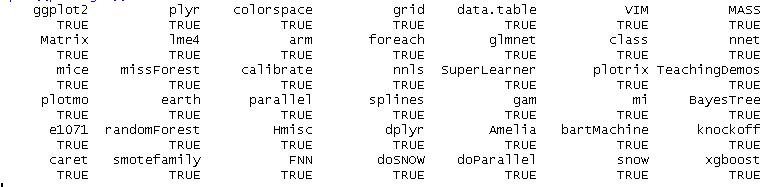ipaktable

# 2 Memory and storage limits to run CBDA

See Memory limits under different OS for various limitations on memory needs while running R. As far as CBDA is concerned, a CBDA object can be up to 200-300 Mb. The default limits are acceptable to run the CBDA algorithm. To know or change the memory allocation, the memory.limit() function reports or increases the limit in force on the total allocation.

``memory.limit(50000)  # to allocate 50Gb of memory``

The function only works on Windows. For Macs , try ulimit -t 600 -v 4000000. This will set the time linmit to 600 seconds and the memory to 4G.

The space needed to save all the workspaces may need to be as large as 1-5 Gb, depending on the number of subsamples. We are working on an new CBDA implementation that reduces the storage constraints.

# 3 Background

This document illustrates the first release of the CBDA protocol as described in the manuscript under review entitled “Controlled Feature Selection and Compressive Big Data Analytics: Applications to Big Biomedical and Health Studies” by Simeone Marino, Jiachen Xu, Yi Zhao, Nina Zhou, Yiwang Zhou, Ivo D. Dinov. University of Michigan, Ann Arbor.

The CBDA protocol has been developed in the R environment. Since a large number of smaller training sets are needed for the convergence of the protocol, we created a workflow that runs on the LONI pipeline environment, a free platform for high performance computing that allows the simultaneous submission of hundreds of independent instances/jobs of the CBDA protocol. The methods, software and protocols developed here are openly shared on our GitHub repository. All software, workflows, and datasets are publicly accessible. The CBDA protocol steps are illustrated in Figure 1.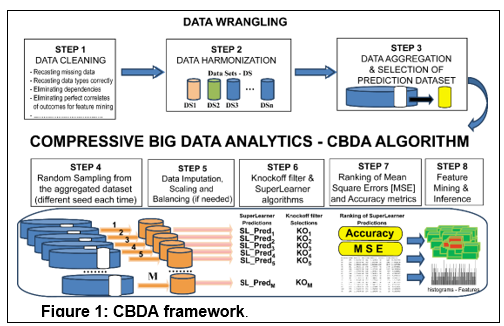This is an introduction to version control and LONI Version Control and LONI.

This is a simple and clear Introduction to the main algorithm used in the CBDA protocol, namely SuperLearner_Intro.

N.B.: This version 1.0.0 is fully tested ONLY on continuous features Xtemp and binary outcome Ytemp.

# 4 Main function of the CBDA package – CBDA()

The CBDA package comprises several functions. The main function is CBDA() that has all the input specifications to run a set M of subsamples from the Big Data [Xtemp, Ytemp]. We assume that the Big Data is already clean and harmonized.

After the necessary data wrangling (i.e., imputation, normalization and rebalancing), an ensemble predictor (i.e., SuperLearner) is applied to each subsample for training/learning.

The list of algorithms (or wrappers) available from the SuperLearner can be displayed by typing listWrappers(). By default, the CBDA package operates with the following algorithms (set with their default values, as described in the SuperLearner package):

• SL.glm: wrapper for generalized linear models via glm()

• SL.xgboost: wrapper that supports the Extreme Gradient Boosting package for SuperLearnering, which is a variant of gradient boosted machines (GBM).

• SL.glmnet: wrapper for penalized regression using elastic net.

• SL.svm: wrapper for Support Vector Machine

• SL.randomForest: wrapper that supports RandomForest

• SL.bartMachine: wrapper that supports bayesian additive regression trees via the bartMachine package

The ensemble predictive model is then validated on a fraction alpha of the Big Data. Each subsample generates a predictive model that is ranked based on performance metrics (e.g., Mean Square Error-MSE and Accuracy) during the first validation step.

# 5 CBDA input specifications

The array of input specifications comprises the following labels (in the square brackets is the default value):

• Ytemp This is the output variable (vector) in the original Big Data

• Xtemp This is the input variable (matrix) in the original Big Data

• label This is the label appended to RData workspaces generated within the CBDA calls [= “CBDA_package_test”]

• alpha Percentage of the Big Data to hold off for Validation [= 0.2]

• Kcol_min Lower bound for the percentage of features-columns sampling (used for the Feature Sampling Range - FSR) [= 5]

• Kcol_max Upper bound for the percentage of features-columns sampling (used for the Feature Sampling Range - FSR) [= 15]

• Nrow_min Lower bound for the percentage of cases-rows sampling (used for the Case Sampling Range - CSR) [= 30]

• Nrow_max Upper bound for the percentage of cases-rows sampling (used for the Case Sampling Range - CSR) [= 50]

• misValperc Percentage of missing values to introduce in BigData (used just for testing, to mimic real cases). [= 0]

• M Number of the BigData subsets on which perform Knockoff Filtering and SuperLearner feature mining [= 3000]

• N_cores Number of Cores to use in the parallel implementation (default is set to 1 core) [= 1, not multicore enabled]

• top Top predictions to select out of the M. This must be < M. [= 1000]

• workspace_directory Directory where the results and workspaces are saved [= getwd()]

• max_covs Top features to include in the Validation Step where nested models are tested [= 100]

• min_covs Minimum number of top features to include in the initial model for the Validation Step. It must be > 2 [= 5]

Sampling ranges for cases (CSR - Cases Sampling Range) and features (FSR - Feature Sampling Range) are then defined as follow:

• FSR = [min_FSR, max_FSR]

• CSR = [min_CSR, max_CSR]

# 6 Secondary CBDA functions

After all the M subsamples have been generated and each predictive model computed, the CBDA function CBDA() calls 4 more functions to perform, respectively:

• CONSOLIDATION (i.e., CBDA_Consolidation()) and ranking of the results where the top predictive models are selected (top) and the more frequent features (BEST) are ranked and displayed as well

• VALIDATION (i.e., CBDA_Validation()) on the top ranked features (i.e., up to “max_covs” number of features) where nested ensemble predictive models are generated in a bottom-up fashion

• Implementation of STOPPING CRITERIA (i.e., CBDA_Stopping_Criteria()) for the best/optimal ensemble predictive model (to avoid overfitting)

• CLEAN UP (i.e., CBDA_CleanUp()) step for deleting unnecessary workspaces generated by the CBDA protocol.

At the end of successfull CBDA() call, 2 workspaces are generated:

• CBDA_input_specs_label_light.RData where most of the results of the M subsamples are saved

• CBDA_input_specs_label_light_VALIDATION.RData where most of the results of the top-ranked subsamples are saved

Throughout the execution of the CBDA protocol, a workspace with the main CBDA specifications is created and loaded whenever necessary (e.g., “CBDA_label_info.RData”).

# 7 CBDA object

A CBDA object is created (e.g., CBDA_object <- CBDA()) with the following data:

• LearningTable: information on the top features selected and the correspondent predictive models’ performances during the learning/training step

• ConfusionMatrices: list of confusion matrices for each of the top nested predictive models in ValidationTable

• SuperLearnerLibrary: list of all the algorithms/wrappers used in the CBDA

• SuperLearnerCoefficients: matrix with all the coefficients generated by the SuperLearner for each subsample and for each algorithms/wrappers used in the CBDA

• ValidationTable: information on the top features selected and the correspondent nested predictive models’ performances

# 8 Example

``````# Set the specs for the synthetic dataset to be tested
n = 300          # number of observations
p = 900          # number of variables

# Generate a nxp matrix of IID variables (e.g., ~N(0,1))
X1 = matrix(rnorm(n*p), nrow=n, ncol=p)

# Setting the nonzero variables - signal variables
nonzero=c(1,100,200,300,400,500,600,700,800,900)

# Set the signal amplitude (for noise level = 1)
amplitude = 10

# Allocate the nonzero coefficients in the correct places
beta = amplitude * (1:p %in% nonzero)

# Generate a linear model with a bias (e.g., white  noise ~N(0,1))
ztemp <- function() X1 %*% beta + rnorm(n)
z = ztemp()

# Pass it through an inv-logit function to
# generate the Bernoulli response variable Ytemp
pr = 1/(1+exp(-z))
Ytemp = rbinom(n,1,pr)
X2 <- cbind(Ytemp,X1)

dataset_file ="Binomial_dataset_3.txt"

# Save the synthetic dataset
write.table(X2,dataset_file,sep=",")

Ytemp <- Data[,1] # set the outcome
original_names_Data <- names(Data)
cols_to_eliminate=1
Xtemp <- Data[-cols_to_eliminate] # set the matrix X of features/covariates
original_names_Xtemp <- names(Xtemp)

workspace_directory <- getwd()

SL.glmnet.0.75 <- function(..., alpha = 0.75,family="binomial"){
SL.glmnet(..., alpha = alpha, family = family)}

simeone <- c("SL.glm","SL.glmnet",
"SL.svm","SL.randomForest","SL.bartMachine","SL.glmnet.0.75")

# Call the Main CBDA function
# Multicore functionality NOT enabled
CBDA_object <- CBDA(Ytemp , Xtemp , M = 16 , Nrow_min = 50, Nrow_max = 70,
top = 15, max_covs = 10 , min_covs = 3)

# Multicore functionality enabled
CBDA_object <- CBDA(Ytemp , Xtemp , M = 24 , Nrow_min = 60, Nrow_max = 80,
N_cores = 4 , top = 20, max_covs = 15 ,  min_covs = 3,
algorithm_list = simeone , label = "CBDA_package_test_multicore")``````

As soon as it is launched, the progress of the CBDA algorithm is shown on the screen (see below).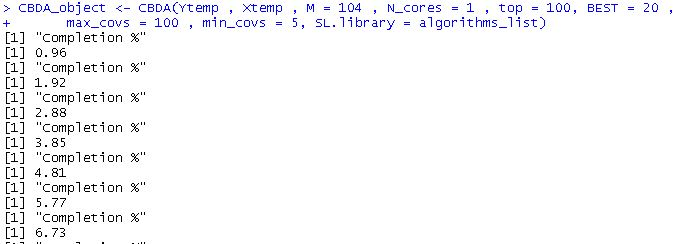After each step of the CBDA algorithm, completion messages and some output is displayed on the screen. For example, after the “CONSOLIDATION” phase is done, the screen shows: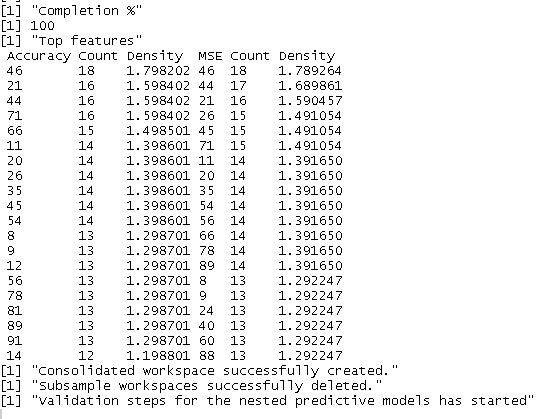Or after the Validation and STopping Criteria steps: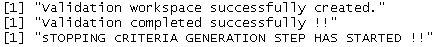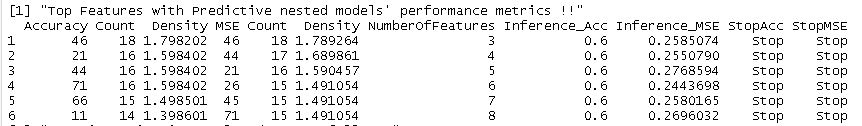If the multicore is enabled, no screen messages are displayed due to the nature of the job allocations.

# 9 Datasets

The CBDA protocol has been applied to 3 different datasets in the manuscript: 2 synthetic ones (Null and Binomial) and a real one (ADNI dataset). The synthetic datasets all have the same number of cases (i.e., 300), but they differ in the number of features. The binomial datasets all have only 10 “true” predictive features, while the null datasets have none.

The Null datasets are organized as follow:

The Binomial datasets are organized as follow:

• Binomial dataset: # of features = 100, with true features (10,20,30,40,50,60,70,80,90,100)

• Binomial dataset 3: # of features = 900, with true features (1,100,200,300,400,500,600,700,800,900)

• Binomial dataset 5: # of features = 1500, with true features (1,100,200,400,600,800,1000,1200,1400,1500).

# 10 LONI pipeline implementation

As mentioned earlier, a large number of smaller training sets are needed for the convergence of the protocol. Thus the CBDA R package has dedicated functions for a workflow implementation that runs on the LONI pipeline environment, a free platform for high performance computing that allows the simultaneous submission of hundreds of simultaneous independent instances/jobs of the CBDA protocol. The main and secondary functions are duplicated and modified to run on the LONI pipeline. The main difference is that they have a job_id parameter that is used to identify the specific job on the server. The functions are CBDA.pipeline(), CBDA_Consolidation.pipeline(), CBDA_Validation.pipeline() and CBDA_Stopping_Criteria.pipeline(). The complete pipeline workflow implementation can be downloaded in this LONI.zip file.

# 11 CBDA complete set of results for the synthetic datasets (Null and Binomial)

Due to the large number of experiments and the many different specification, the complete set of results for the 3 binomial datasets are shown at the following links:

The complete set of results for the histograms in Figure 3 are shown at the following links:

The complete set of results shown in the next figure (i.e., Figure 2 of the manuscript under review) are here.

The figure below shows a set of heatmaps and histograms to summarize all the experiments in the manuscript under review